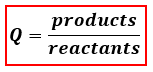# Problem: For the reaction below Kc = 168C(s) + CO2(g) ⇌ 2CO(g)A mixture contains some C(s), [CO] = 0.50 M and [CO 2] = 0.75 M. Therefore the system willa) Shift to produce more C (s) and CO2 (g) because Q &lt; K.b) Shift to produce more CO because Q &lt; K.c) Shift to produce more C (s) and CO2 (g) because Q &gt; K.d) Shift to produce more CO because Q &gt; K.e) The system is already in equilibrium

###### FREE Expert Solution
87% (184 ratings)
###### FREE Expert Solution

We are being asked to identify the shift produced in the system with the given concentrations for CO2 and CO. We will use the reaction quotient, Q, to determine if the chemical reaction would be at equilibrium or not. The formula for Q is:Depending on if Q is greater than or less than K, our reaction will shift to attain equilibrium by reaching the equilibrium constant K:

87% (184 ratings)###### Problem Details

For the reaction below Kc = 168

C(s) + CO2(g) ⇌ 2CO(g)

A mixture contains some C(s), [CO] = 0.50 M and [CO 2] = 0.75 M. Therefore the system will

a) Shift to produce more C (s) and CO2 (g) because Q < K.

b) Shift to produce more CO because Q < K.

c) Shift to produce more C (s) and CO2 (g) because Q > K.

d) Shift to produce more CO because Q > K.

e) The system is already in equilibrium

What scientific concept do you need to know in order to solve this problem?

Our tutors have indicated that to solve this problem you will need to apply the The Reaction Quotient concept. You can view video lessons to learn The Reaction Quotient. Or if you need more The Reaction Quotient practice, you can also practice The Reaction Quotient practice problems.

What is the difficulty of this problem?

Our tutors rated the difficulty ofFor the reaction below Kc = 168C(s) + CO2(g) ⇌ 2CO(g)A mixtu...as low difficulty.

How long does this problem take to solve?

Our expert Chemistry tutor, Jules took 2 minutes and 11 seconds to solve this problem. You can follow their steps in the video explanation above.

What professor is this problem relevant for?

Based on our data, we think this problem is relevant for Professor Cubonova's class at OSU.